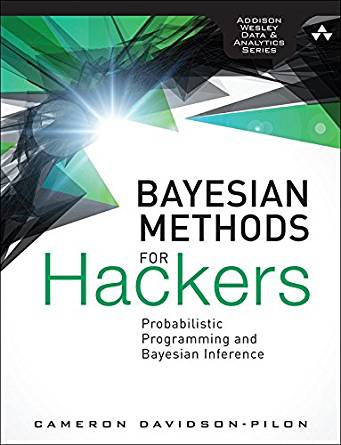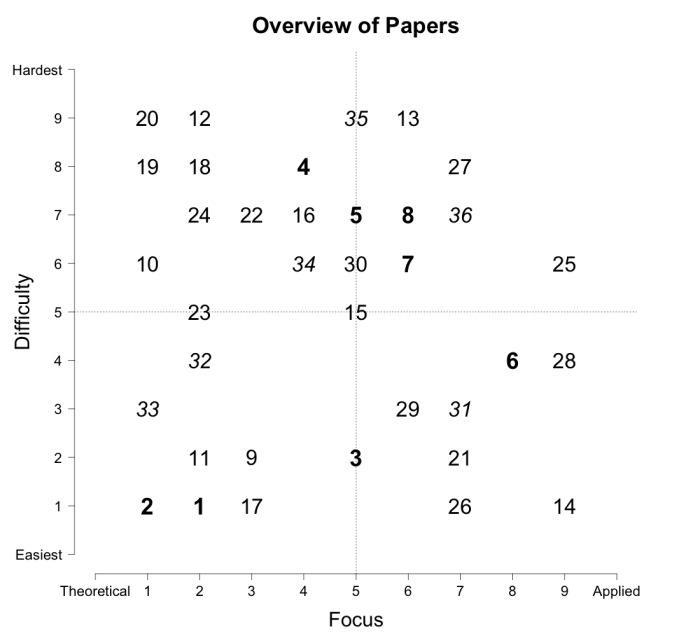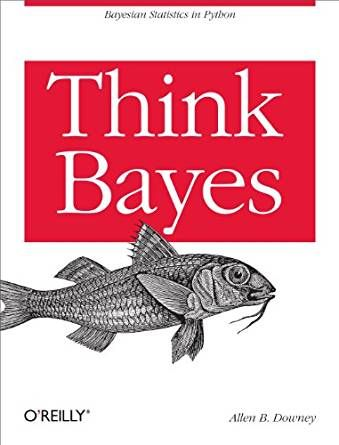"/> Learn Bayesian methods in 4 steps - by reading and by doing

# Learn Bayesian methods in 4 steps - by reading and by doing

This post proposes a 4-step path for learning Bayesian methods. The first step is going through the book: "Bayesian methods for hackers", second, using complementary books for probability and statistics, the third, reading How to become a Bayesian in eight easy steps: and last, going through the book full of exercises: "Think Bayes".

## Background

When reading this article you probably have some experience with machine learning models. You might have tried RandomForest, XGBoost, etc. They are easy to use but it is difficult to understand how final predictions were done. This is sometimes referred to as predictions out of the black box. Of course, there are techniques that might help a bit e.g. extracting feature importance from the trained models.

Bayesian methods are a great helper in understanding how actual reasoning was done. These methods provide probabilistic models that can describe the process that produced the data we want to process. Besides accurate predictions, we often need an understanding of what is important in the process. Through understanding gain confidence in used models. We need convenience when moving with machine learning from toy projects to real business applications. Bayesian can offer such understanding and convenience and that is why these methods are gaining attention.

In this blog post I will present 4 steps for Bayesian methods mastery. The rough estimate is that you will need to dedicate around 100 hours to complete this 4-steps path.

## 1. "Bayesian methods for hackers" - free book in form of Jupyter notebooks with interactive content.The first chapter of "Bayesian methods for hackers" (BMH) will introduce you to the Bayesian way of thinking. Understand reducing uncertainty using observations. You will go through the first example that is showing statistical modeling of the texting rate. The following chapters explain new techniques in detail. New techniques are immediately applied to solving exemplary problems.

For myself, when progressing through the book, I felt that I need to refresh my statistical knowledge and started looking for the proper book. The math required to use these methods is already provided in the book. Yet, I needed a better understanding of different random variable distributions. This is something that I already learned years ago in university courses but I needed a refresher.

## 2. Probability and Statistics books that will help you learn/refresh math to build a solid foundation.

My choice for complementary probability and statistics books was twofold:

## 3. "How to become a Bayesian in eight easy steps: An annotated reading list".

"How to become a Bayesian in eight easy steps: An annotated reading list" by Etz, Alexander, et al., is a paper, not a target at Computer Scientists. Actually, it originates from the field of psychology but is written in a domain-agnostic style, so readers from any discipline can enjoy reading this. The paper has a survey style and uses the classification of the covered papers in two dimensions: difficulty (from easy to hard), and focus (from theoretical to practical). See the Figure below, borrowed from the paper.The main paper and references are rather light reading and I found it useful in building context for diving into Bayesian analysis.

## 4. Exercises to develop Bayesian thinking: "Think Bayes" by Allen Downey.Another great book to learn Bayesian thinking. It is divided into smaller units than BMH which makes it easier to digest for readers that are quickly losing attention when reading scientific stuff. When compared to BMH, it has much more examples. Crashing a large number of cases is to me very good approach for training Bayesian intuition and learning methods.

Will you give it a try to Bayesian methods? If you have a proposal for an alternative learning path - please email me.

Any comments or suggestions? Let me know.

```@article{Saf2019Learn,